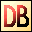DelphiBasicsTFloatFormatType Formats for use in floating point number display functions SysUtils unit
 type TFloatFormat = (ffGeneral, ffExponent, ffFixed, ffNumber, ffCurrency);
Description
The TFloatFormat type is used by the following SysUtils functions :

CurrToStrF? For displaying currency values FloatToStrF For general float displaying FloatToText For formatting to an array

The possible values of TFloatFormat are :

ffGeneral

Defines general number formatting that aims to keep the resultant value as compact as possible. It removes trailing zeros and the decimal point where appropriate. No thousand separators are shown. Exponent format is used if the mantissa is too large for the specified Precision value of the formatting command. In this case, the Digits value (0..4) determines the minimum number of exponent digits shown. The decimal point character is determined by the DecimalSeparator variable.

ffExponent

Commonly referred to as the Scientific or Engineering format, the exponent refers to the letter E followed by a number. The number gives the power of 10 of the number. For example, E+15 means 1015. The exponent always has a + or - sign. This exponent is preceded by a number that always has one digit before the decimal place.

For example : 123.456 formats as 1.23456E+2 0.00123 formats as 1.23E-3

The using function Precision parameter gives the number of displayed digits before the E, and the Digits parameter gives the number (0..4) of digits after the E.

The decimal point character is determined by the DecimalSeparator variable.

ffFixed

This format again uses no thousands separator. It displays Precision digits before the decimal point, and Digits digits after. If there are too many digits before the decimal point, then the Exponent format is used instead.

The decimal point character is determined by the DecimalSeparator variable.

ffNumber

Same as ffFixed, except that thousand separators are used. These are defined by the ThousandSeparator variable.

ffCurrency

Same as ffNumber, but with a currency symbol (string) added, as defined by the CurrencyString variable. Additionally, the formatting is influenced by the CurrencyFormat and NegCurrFormat variables.
Notes
Note above that the Precision and Digits values of the function using TFloatFormat depend on the format chosen.
Related commands
 CurrencyDecimals Defines decimal digit count in the Format function CurrencyFormat Defines currency string placement in curr display functions CurrencyString The currency string used in currency display functions CurrToStrF Convert a currency value to a string with formatting DecimalSeparator The character used to display the decimal point FloatToStrF Convert a floating point value to a string with formatting NegCurrFormat Defines negative amount formatting in currency displays ThousandSeparator The character used to display the thousands separatorDownload this web site as a Windows program.